AnyLogic

# geometric

 Probability mass function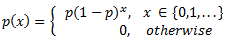Distribution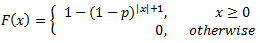Mean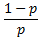Variance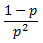Mode 0

The Geometric distribution is a discrete distribution bounded at 0 and unbounded on the high side. It is a special case of the Negative Binomial distribution. In particular, it is the direct discrete analog for the continuous Exponential distribution. The Geometric distribution has no history dependence, its probability at any value being independent of a shift along the axis.

The Geometric distribution has been used for inventory demand, marketing survey returns, a ticket control problem, and meteorological models.

#### Examples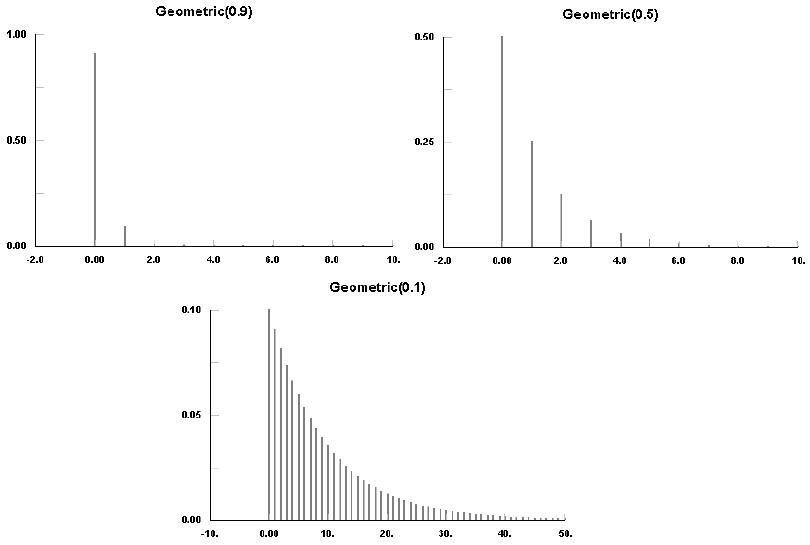### geometric(double p)

Description
Generates a sample of the Geometric distribution.
Parameters
Name Type of value Description
p double The probability of occurrence.
Result
Type Description
int The generated sample.

### geometric(double p, java.util.Random r)

Description
Generates a sample of the Geometric distribution using the specified random number generator.
Parameters
Name Type of value Description
p double The probability of occurrence.
r java.util.Random The random number generator.
Result
Type Description
int The generated sample.

This document includes content from the “Stat::Fit User’s Manual”. Copyright 2016 Geer Mountain Software Corp.